# Comparison of Different Methods to Evaluate Measurement Uncertainty in Bimetallic Thermometers Used in Industrial Equipment

Title: Comparison of Different Methods to Evaluate Measurement Uncertainty in Bimetallic Thermometers Used in Industrial Equipment
José Daniel Hernández-Vásquez, Andrés Leonardo Jutínico-Alarcon, Emanuel Alberto Simanca-Celedon, Alejandro Romero Acuña, Willman Orozco-Lozano, Mercy Villate-Fonseca, Manuel J. Campuzano

Corresponding email:

Hernández-Vásquez, J.D., Jutínico-Alarcon, A.L., Simanca-Celedon, E.A., Romero Acuña, A., Orozco-Lozano, W., Villate-Fonseca, M., Campuzano, M.J., 2023. Comparison of Different Methods to Evaluate Measurement Uncertainty in Bimetallic Thermometers Used in Industrial Equipment. International Journal of Technology. Volume 14(2), pp. 374-386

188
 José Daniel Hernández-Vásquez Universidad Antonio Nariño. Faculty of Mechanical, Electronic and Biomedical Engineering (FIMEB) Andrés Leonardo Jutínico-Alarcon Universidad Antonio Nariño. Faculty of Mechanical, Electronic and Biomedical Engineering (FIMEB) Emanuel Alberto Simanca-Celedon Universidad Antonio Nariño. Faculty of Mechanical, Electronic and Biomedical Engineering (FIMEB) Alejandro Romero Acuña FIMEB / Universidad Antonio Nariño Willman Orozco-Lozano FIMEB / Universidad Antonio Nariño Mercy Villate-Fonseca Universidad Antonio Nariño. Faculty of Mechanical, Electronic and Biomedical Engineering (FIMEB) Manuel J. Campuzano Universidad Antonio Nariño. Faculty of Industrial Engineering
Email to Corresponding Author

Abstract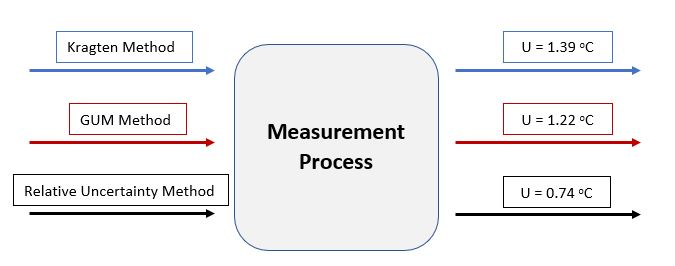This paper compares different methods (Kragten, Guide to the expression of uncertainty in measurement –GUM– and Relative Uncertainty) to evaluate the measurement uncertainty in bimetallic thermometers. The motivation for the development of the work is based on a need detected in the industry to increase the metrological reliability of thermometers for temperature control in the most diverse measurement processes. The applied methodology is based on the concepts enshrined in the classical literature about the GUM, Kragten and Relative methods. The consolidated results confirmed that, for temperature measurement applications, the GUM method is more appropriate for estimating the measurement uncertainty. The value obtained was equal to 1.22oC throughout the calibration range of the instrument. In conclusion, this work showed that an increase in metrological reliability for the measurement of temperature, fundamental magnitude in industrial processes, can be obtained by evaluating different methods for estimating the measurement uncertainty.

GUM; Kragten; Measurement uncertainty; Relative uncertainty; Thermal metrology

Introduction

The estimation of the uncertainty associated with the measurement is a fundamental task that produces technical-economic gains for the area of Mechanical Engineering. At an industrial level, specifically in temperature measurement, it is strategic to know the parameter that best represents the dispersion of a measurand within a certain confident level, i.e. measurement uncertainty.
The specialized literature confirms that the metrological reliability of a measurement system is intimately related to the estimated value of its uncertainty (Wang et al., 2018). In addition, different studies (Golijanek-J?drzejczyk et al., 2019; Farrance, Badrick, and Frenkel, 2018; Gnauert et al., 2018; Farrance and Frenkel, 2012) show that an improvement in the factors that influence the measurement uncertainty tends to increase the availability of measuring equipment and, consequently, an increase in the quality of manufactured products, according to guidelines specified in ISO 9001:2015 (International Organization for Standardization, 2015).

Thus, according to the literature, developing and investigating different methods for the estimation of measurement uncertainty is a challenge for engineering and measurement science. Typically, the guidelines defined in the GUM (Guide for the expression of measurement uncertainty) (JCGM, 2008) are widely applied by several Metrology Laboratories in calculating measurement uncertainty. Despite its application, this method presents some inconsistency: (i) it combines different probability distributions (e.g., normal, rectangular, triangular); (ii) it considers a Gaussian distribution for the experimental data of the calibration.

In practice, these limitations contribute to a deviation between the physical nature of the problem and the mathematical method adopted forits analysis. For the specific case of the calibration of instruments for the measurement of temperature at an industrial level, it is not very feasible from a technical and economic point of view to calibrate all the points along the instrument scale. For example, a bimetallic thermometer with a scale from 0 to 120oC that carries out 120 calibration points (assuming that it advances from 1oC to 1oC) and, additionally, perform 10 repetitions at each point, would generally total 1200 experimental points. This situation would be ideal to reduce the uncertainty associated with the measurement. However, each calibration point has a duration of approximately 2 hours due to the thermal stability of the standard measurement instrument and the medium used for generating heat. In this way, to carry out the entire process, approximately 2400 hours, which means 100 continuous days of measurement, are required. Clearly, for the industrial sector, this scenario is unfeasible from a technical point of view and, logically, from an economic point of view. For this reason, in practice, a few calibration points (from 1 to 3 experimental points) are evaluated according to the NT VVS 103 standard (Thermometers, Contact, Direct Reading: Calibration, 1994) for the calibration of thermal sensors instruments (RTDs, Thermocouples, and Glass Thermometers). Thus, the hypothesis is assumed that the experimental data follow a normal distribution of probability. Under this hypothesis, the GUM method is applied to calculate the uncertainty associated with the measurement, obtaining results with deviations in terms of the physical nature of the problem. To counteract the effects of this situation, the specialized literature presents some alternative methods for analyzing measurement uncertainty. Among the methods, the ISO GUM Supplement 1, known as the Monte Carlo method (JCGM, 2008), the Kragten method, and applications of Bayesian statistics, among others, stand out. The literature presents various works where the potential of the GUM, Monte Carlo, and Kragten methods have been compared Zarate et al. (2022), Aro et al. (2021), Khan and Ibrayeva (2020), Cremona et al. (2018), Sardjono and Wijonarko (2018), Horsky, Irrgeher, and Prohaska (2016), Guerrasio et al. (2013), Theodorou, Zannikou, and Zannikos (2012), resulting in a  robust Monte Carlo and, additionally, similar results between the GUM and Kragten methods. However, in ??thermal metrology applied to the industrial sector, the literature does not show a direct comparison between the GUM and Kragten methods. Additionally, due to its ease of application, the relative uncertainty method could be adopted without due consideration, obtaining inbetter results. However, they lack metrological reliability because the formulation of the method does not represent the physical nature of the problem.

In this order of ideas, this work seeks to compare three of the most used methods for calculating measurement uncertainty: ISO GUM, the Kragten method, and the relative uncertainty method. Discuss its advantages, disadvantages, limitations, and considerations for its application in the calibration of bimetallic-type temperature measurement instruments, which are widely used in the industrial sector.

Theoretical Fundament

To perform an analysis of the experimentally obtained data, this section summarizes the main concepts associated with outlier analysis, as well as the methods adopted for the analysis and calculation of the uncertainty associated with the measurement, i.e., GUM, Kragten's, and relative method. In relation to the application of a polynomial model that allows for establishing the adjustment equation in a measurement system, the reference (Esraa et al., 2022) shows more details about this procedure. In this order of ideas, this work seeks to compare three of the most used methods for calculating measurement uncertainty: ISO GUM, the Kragten method, and the relative uncertainty method. Discuss its advantages, disadvantages, limitations, and considerations for its application in the calibration of bimetallic-type temperature measurement instruments, which are widely used in the industrial sector.

2.1. Analysis of outliers

Once the experimental data were consolidated, the results analysis methodology was applied, starting with the application of the Chauvenet method (Wang, Caja, and Gómez, 2018) for the elimination of outliers. According to this criterion, a measure must be eliminated if: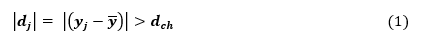where  is the distance between an experimental point ; and  denotes the mean of the sample;  denotes the limit value for elimination by the Chauvenet criterion, defined by Equation (2):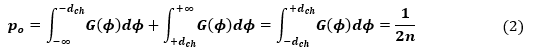where  is the probability of rejecting a value that is considered as outliers,  is a Gaussian function. In other words, it specifies that an experimental value can be eliminated if the probability of obtaining a certain deviation from the mean is less than 1/2n. This criterion states that if the value of    calculated by Equation (3) is greater than the value of   tabulated for the Chauvenet criterion, a measured value   must be eliminated (Wang, Caja, and Gómez, 2018).

2.2. Uncertainty Analysis

2.2.1. Uncertainty Analysis: GUM Method

Following the GUM methodology described in section 2.1, four sources of uncertainty were considered:

• ·  Instrument resolution (uinst) is associated with rectangular probability distribution, according to defined in GUM (JCGM, 2008) for analogical instruments. Thus, the resolution of instrument (2oC) is divided by .
• ·  Reference instrument (up) is associated with the calibration of the reference instrument. This value is consigned in the Certificate of Calibration. The expanded uncertainty equals 0.095oC, and the de coverage factor is k = 2. Thus, the standard uncertainty is 0.0475oC.
• ·   Repeatability (ur) is associated with different measurements realized in the experiments. In order to calculate this source of uncertainty, Equation (4) was applied from the experimental data.
• ·    Polynomial adjustment (us) is associated with less uncertainty of polynomial. The results confirmed that this value equals 0.1522oC for a polynomial of degree two.

Equation (4) was used to calculate the combined uncertainty (uc) from the contributing sources of uncertainty: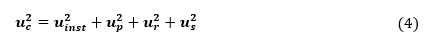The coverage factor, determined from a t-student distribution, corresponds to k = 2 for all the experimental points. Thus, the expanded uncertainty (UE) associated with the temperature measurement for a confidence level (?) of 95.45% was determined by applying Equation (5). Table 6 summarizes the results of uncertainty analysis.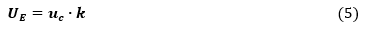2.2.2. Uncertainty Analysis: Kragten's Method

From the sources of uncertainties determined by the GUM method, Kragten's method is applied. Equation (6) denotes the measurand used to determine the corrected systematic error (Ec ).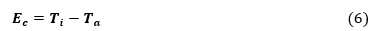The uncertainties associated with the temperature indicated by the instrument ( Ti) and that associated with the temperature adjusted by the interpolating polynomial (Ta ) are determined by Equations (7) and (8).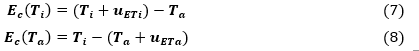Equations (9) and (10) were used to calculate the deviations associated with the temperature indicated by the instrument (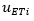) and adjusted by the interpolating polynomial (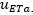).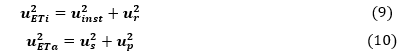In the sequence, Equations (11) and (12) were applied to estimate the uncertainties associated with the temperature indicated by the instrument  and adjusted by the interpolating polynomial .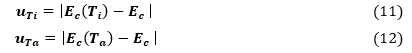Finally, the combined uncertainty is calculated by applying Equation (13), and the expanded uncertainty (k = 2) was determined by applying Equation (14).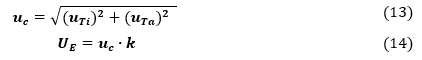2.2.3. Uncertainty Analysis: Uncertainty Relative Method

In relation to the relative uncertainty method, Equation (6) shows the expression that represents the measurand. Equations (15) and (16) estimate the measurement uncertainty.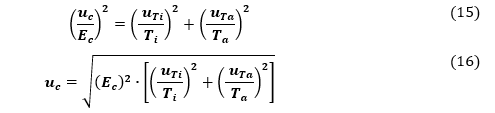Experimental Methods

The experimental methodology is described in this section. In the course of the experiments performed in a metrology laboratory, under controlled environmental conditions, a bimetallic thermometer was calibrated (Manufacturer: Rockage; Model: SHB-05; Range: -20oC to 120oC; Resolution: 1oC) using the method of direct comparison. This calibration was performed using a standard dry block as a means for generating heat (Manufacturer: Reed BX-150, Temperature measurement range: 27.0oC to 350.0oC) and a thermocouple with a digital temperature indicator (Manufacturer: Xintest, Model: HT-9815 Thermocouple Thermometers, ID: EPS-001, Serial Number: 201703021366, Range: 0.0oC to 99.9oC). Subsequent sections detail the technical characteristics of the experimental apparatus and a description of the procedure for collecting experimental data in the laboratory.

The Rockage bimetallic thermometer calibration experiments were performed in a Metrology Laboratory prepared for that purpose, following the guidelines established in the normative document NT-VVS-103:1994, Thermometers contact direct Reading: Calibration. It is important to note that a conventional Metrology Laboratory, when performing this type of calibration, generally takes of 3 experimental points along the range of the instrument. In addition, it does not perform repetitions at each point. As an added value to the research and considering the relevance of the measurement of temperature in equipment and measurement processes in the industry, in this work, we chose to be more rigorous with obtaining the experimental data and its subsequent statistical treatment. In relation to the calibrated experimental points, 8 of these were taken throughout the range of indicators of the instrument, varying from 30oC to 100oC. In addition, for each calibration point 6 repetitions were taken for the instrument (bimetal thermometer) and 6 for the measurement pattern, totaling 12 experimental points in each measurement. Throughout the experiment, 96 points were evaluated in 12 continuous hours of measurement.

Finally, the experimental data allowed to evaluate the metrological reliability of the bimetallic thermometer, consolidated in Table 1. This table highlights in red some values considered as possible outliers.

Table 1 Experimental data of the calibration in the laboratory

 Reference temperature in the Dry Block Instrument (I) / Reference (R) Temperature Measurements Average Standard Desviation #1 #2 #3 #4 #5 #6 °C °C °C °C °C °C °C °C °C 30 I 30 29 30 30 31 30 30 0.6325 R 30.6 30.4 30.4 30.3 30.2 30.2 30.4 0.1517 40 I 39 40 40 39 39 41 40 0.8165 R 39.1 39.3 39.3 39.2 39.2 39.3 39.2 0.0816 50 I 50 50 50 50 49 50 50 0.4082 R 49.5 49.5 49.5 49.5 49.4 49.5 49.5 0.0408 60 I 61 61 61 60 60 61 61 0.5164 R 60.2 60.2 60.2 60.4 60.3 60.2 60.3 0.0837 70 I 70 70 70 69 69 70 70 0.5164 R 69.5 69.6 69.6 69.6 69.6 69.5 69.6 0.0516 80 I 80 79 79 79 80 80 80 0.5477 R 79.7 79.7 79.7 79.9 79.9 79.9 79.8 0.1095 90 I 89 90 90 90 89 90 90 0.5164 R 88.6 88.6 88.6 88.6 88.5 88.5 88.6 0.0516 100 I 100 99 99 99 100 100 100 0.5477 R 99.7 99.6 99.6 99.7 99.7 99.6 99.7 0.0548

This hypothesis is based on the observation of the experimental data collected for each experimental point, since they are more dispersed when compared to the other 5 data collected. However, this hypothesis must be confirmed by applying the Chauvenet method, as evidenced in the results section.

Results and Discussion

This section consolidates the main results of the investigation. It was divided into three large blocks to facilitate its development: (i) Analysis of outliers. In this stage, the parametric technique proposed by Chauvenet will be applied to eliminate aberrant values, i.e., outliers. The reason why these values ??are produced is very varied. Consequently, the uncertainty associated with the temperature measurement increases and unquestionably, the impact of a given measurement process will be negative both technically and economically. Hence, the importance of carrying out this important stage within the proposed methodology; (ii) Estimation of the adjustment polynomial. By applying the ordinary least squares method, it is expected to estimate a polynomial that best represents the physics of the problem studied. This polynomial is associated with the least adjustment uncertainty, also known as the quadratic mean deviation. In addition to allowing the obtaining of adjusted temperature values, the polynomial allows obtaining errors and uncertainties for any indication in the calibration range, even in those points where it was not possible to perform the experimental calibration; (iii) Application of techniques to estimate uncertainty. In this stage, the enshrined theory was studied in detail for three uncertainty estimation techniques: GUM, Kragten, and Relative Uncertainty. Subsequently, the experimental data analysis was performed to establish the expanded uncertainty associated with the temperature measurement, as well as the associated to systematic errors.

4.1.  Analysis of Outliers

It is emphasized that the value of  is a function of the number of measurements taken. By applying Equation (3) and using the data in Table 1 it was possible to obtain the value of  (calculated) for each experimental point. In addition, the value of  (critical) was obtained by applying Equation (2). Table 2 summarizes the calculation for  and shows the value of .

Table 2 Calculation of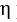parameter and the  value

 Instrument (I) / Reference (R) Calculation of ? from the Temperature Measurements Critical value of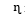c123456 I 0.0 1.6 0.0 0.0 1.6 0.0 1.73 R 1.6 0.3 0.3 0.3 1.0 1.0 I 0.8 0.4 0.4 0.8 0.8 1.6 R 1.6 0.8 0.8 0.4 0.4 0.8 I 0.4 0.4 0.4 0.4 2.0 0.4 R 0.4 0.4 0.4 0.4 2.0 0.4 I 0.6 0.6 0.6 1.3 1.3 0.6 R 0.6 0.6 0.6 1.8 0.6 0.6 I 0.6 0.6 0.6 1.3 1.3 0.6 R 1.3 0.6 0.6 0.6 0.6 1.3 I 0.9 0.9 0.9 0.9 0.9 0.9 R 0.9 0.9 0.9 0.9 0.9 0.9 I 1.3 0.6 0.6 0.6 1.3 0.6 R 0.6 0.6 0.6 0.6 1.3 1.3 I 0.9 0.9 0.9 0.9 0.9 0.9 R 0.9 0.9 0.9 0.9 0.9 0.9

Table 2 highlights in red the  c values calculated for the three temperature points suspected of being outlier values identified in Table 1. The results of the table above confirmed that three measurements of temperature must be eliminated because the eta parameter calculated is major than the critical value.

These values () were highlighted in red color (Table 2), and the correspondent temperature values were highlighted in red color (Table 1). These values correspond to measures 5 of the instrument (49oC) and reference instrument (49.4oC), whose reference value of the dry block is 50oC, as well as measure 4 of the reference instrument (60.4oC) whose value of the dry block reference is 60oC. Once the mean and the standard deviation were recalculated for those temperature values where the outliers were eliminated, it was found that the standard deviation decreased when compared with the standard deviation of the original data.

4.2. Estimation of the Adjustment Polynomial

In the sequence of the analysis and using the experimental data, without considering the outliers, it was possible to establish a mean value that represents the total of the measurements obtained for each experimental point. Thus, Table 3 consolidates the average values for the bimetallic thermometer (instrument) and the reference instrument. Likewise, the value of the systematic error is shown. This value is obtained by the difference between the temperature indicated by the instrument and the temperature of the reference instrument, as established by the International Vocabulary of Metrology (VIM) (JCGM, 2012). According to the International Vocabulary of Metrology (VIM) (JCGM, 2012), the value that represents the discrepancy between the temperature measured by an instrument and the temperature measured by a reference instrument is defined as the difference between the two readings.

Table 3 Average temperature and calculation of systematic error

 Average temperature of the instrument (Ti) Average temperature of the reference instrument (Tp) Systematic error (E) °C °C °C 30 30.4 -0.4 40 39.2 0.8 50 49.5 0.5 61 60.2 0.8 70 69.6 0.4 80 79.8 0.2 90 88.6 1.4 100 99.7 0.3

Using the values of Ti and Tp, it was possible to determine the interpolating polynomial that best represents the physical nature of the experiment by applying the ordinary least squares method. The objective is to identify the best polynomial and the uncertainty of adjustment associated with each polynomial (us) must be determined. The polynomial with the smallest (us) is considered to be the best representation of the experimental physical model. Regarding the number of degrees of freedom, this parameter is calculated by the difference between the number of possibilities (number of experimental points n) and the number of restrictions imposed (in the case of using fit polynomials, the number of restrictions is given by a number of coefficients of the polynomial). Table 4 consolidates the results obtained for the adjusted temperature, applying each polynomial, and the table 5 shows the final value for the adjustment uncertainty associated with each polynomial.

Table 4 Adjusted Temperature (Ta) for each polynomial

 Polynomial of Degree 1 Polynomial of Degree 2 Polynomial of Degree 3 °C °C °C 29.8 30.1 30.2 39.7 39.8 39.8 49.6 49.5 49.7 60.5 60.4 60.9 69.5 69.3 70.2 79.4 79.3 80.8 89.3 89.4 91.4 99.2 99.6 102.1

Table 5 Calculation of the uncertainty associated with the polynomial adjustment (us)

 Polynomial of Degree 1 Polynomial of Degree 2 Polynomial of Degree 3 °C °C °C 0.1568 0.1522 0.2826

The result above shows as the lower value of uncertainty is 0.1522oC and corresponds to the polynomial of degree two. Thus, this result confirmed that, contrary to the expected, a polynomial of degree two models the physical nature of the problem with an uncertainty less than that associated with a polynomial of the first degree. Thus, in this procedure, it was evident that the best interpolating polynomial of adjustment corresponds to a second-degree polynomial under the following equation: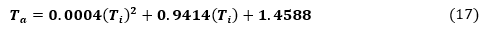In this expression : denotes the temperature set by the interpolating polynomial and : denotes the temperature indicated by the instrument.

Therefore, it is good calibration practice to test at least three degrees of a polynomial to define the one that offers the lowest adjustment uncertainty and, therefore, the lowest uncertainty associated with the measurement. The evaluation of a polynomial of the fourth degree or higher, although it offers a better result from a mathematical point of view (i.e.: smaller fit errors), does not, however, represent the physical nature of the phenomenon studied.

The application of a second-degree interpolating polynomial allows for correcting the experimental results measured by the measuring instrument, thus eliminating the systematic error inherent to the measurement process, and facilitating the process of incorporating calibration in the remote data processing.

4.3. Application of Techniques to Estimate Uncertainty

Once the uncertainty of the adjustment is determined, we estimate the expanded uncertainty associated with the measurement by the three methods under study: GUM, Kragten, and Relative Uncertainty.

4.3.1. Uncertainty Analysis: GUM Method

In the table above it is possible to identify that the greatest source of uncertainty is associated with the resolution of the measurement instrument, while repeatability is the source of the lowest contribution.

Table 6 Uncertainty analysis: GUM method

 Uncertainty associated to instrument resolution (uinst) Uncertainty associated to reference instrument (up) Uncertainty associated to repeatability (ur) Uncertainty associated to Polynomial Adjustment (us) Combined Uncertainty (uc) Coverage Factor (k) Expanded Uncertainty (UE) Confidence Level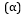°C °C °C °C °C - °C % 0.5774 0.0475 0.0619 0.1522 0.6022 2.0 1.22 95.45% 0.5774 0.0475 0.0333 0.1522 0.5999 2.0 1.22 0.5774 0.0475 0.0000 0.1522 0.5990 2.0 1.21 0.5774 0.0475 0.0183 0.1522 0.5992 2.0 1.22 0.5774 0.0475 0.0211 0.1522 0.5993 2.0 1.22 0.5774 0.0475 0.0447 0.1522 0.6006 2.0 1.22 0.5774 0.0475 0.0211 0.1522 0.5993 2.0 1.22 0.5774 0.0475 0.0224 0.1522 0.5994 2.0 1.22

Table 7 consolidates the final results of the method. It shows the temperature set by the polynomial, as well as the corrected systematic error module. This parameter was determined by calculating the difference between the temperature indicated by the instrument and the temperature set by the interpolating polynomial. In addition, the expanded uncertainty associated with the temperature measurement is shown, and finally, the calculation of the total error of the measurement: Total Error =|Systematic error|+|Expanded uncertainty|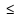(Process tolerance).

Table 7 Uncertainty analysis: GUM method

 Adjusted Temperature (Ta) Correction of Systematic Error Module (|Ec|) Expanded Uncertainty (UE) Error Total (ET =|Ec|+ |UE|) °C °C °C °C 30.0 0.1 1.22 1.3 40.0 0.2 1.22 1.5 50.0 0.5 1.21 1.7 61.0 0.6 1.22 1.8 70.0 0.7 1.22 1.9 80.0 0.7 1.22 1.9 90.0 0.6 1.22 1.8 100.0 0.4 1.22 1.6

From the Table 7 it is observed that the expanded uncertainty associated with the temperature measurement applying the GUM method is equal to 1.22oC in all the calibration points except for point 50oC, where an expanded uncertainty equal to 1.21 was estimated.

4.3.2. Uncertainty analysis: Kragten's method

Table 8 consolidates the results of the uncertainty analysis by Kragten's method. It can be observed in this table that, unlike the GUM method, the uncertainty is not constant throughout the instrument's measurement range, but varies from 1.21oC to 1.39oC.

Table 8 Consolidated results: Kragten's method

 (Ec) [Ec (Ti )] [Ec (Ta )] (uTi) (uTa) (uc) (k) (UE)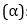°C °C °C °C °C °C - °C % -0.1 0.6 -0.2 0.6 0.2 0.6523 2.0 1.32 95.45% 0.2 0.9 0.1 0.7 0.2 0.6855 2.0 1.39 0.5 1.0 0.3 0.6 0.2 0.5990 2.0 1.21 0.6 1.2 0.5 0.6 0.2 0.6350 2.0 1.29 0.7 1.3 0.5 0.6 0.2 0.6350 2.0 1.29 0.7 1.3 0.5 0.6 0.2 0.6393 2.0 1.30 0.6 1.2 0.4 0.6 0.2 0.6350 2.0 1.29 0.4 1.0 0.2 0.6 0.2 0.6393 2.0 1.30

(Ec): Correction of Systematic Error; [Ec (Ti )]: Correction of Systematic Error associated to Indicated temperature; [Ec (Ta )]: Correction of Systematic Error associated to Adjusted temperature; (uTi): Uncertainty associated to Indicated temperature; (uTa): Uncertainty associated to Adjusted temperature; (uc): Combined uncertainty; (k): Coverage factor; (UE): Expanded Uncertainty;: Confident level.

4.3.3. Uncertainty Analysis: Uncertainty Relative Method

Table 9 consolidates the results of the uncertainty analysis by the Relative Uncertainty method. It can be observed that, unlike the GUM and Kragten's method, the uncertainty is much lower when compared to that obtained by the previous methods. This uncertainty varies from 0.0025oC to 0.012oC.

Table 9 Consolidated results: Uncertainty relative method

 (Ec) (Ti ) (Ta ) (uTi) (uTa) (uc) (k) (UE)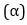°C °C °C °C °C °C - °C % -0.1 30.0 30.1 0.6 0.2 0.0012 2.0 0.0025 95.45% 0.2 40.0 39.8 0.6 0.2 0.0037 2.0 0.0074 0.5 50.0 49.5 0.6 0.2 0.0056 2.0 0.011 0.6 61.0 60.4 0.6 0.2 0.0061 2.0 0.012 0.7 70.0 69.3 0.6 0.2 0.0058 2.0 0.012 0.7 80.0 79.3 0.6 0.2 0.0050 2.0 0.010 0.6 90.0 89.4 0.6 0.2 0.0038 2.0 0.0077 0.4 100.0 99.6 0.6 0.2 0.0024 2.0 0.0049

(Ec): Correction of Systematic Error; (Ti ): Indicated temperature by the instrument;  (Ta ): Adjusted temperature by polynomial; (uTi): Uncertainty associated to Indicated temperature; (uTa): Uncertainty associated to Adjusted temperature; (uc): Combined uncertainty; (k): Coverage factor; (UE): Expanded Uncertainty;: Confident level.

4.4. Metrological Comparison of the GUM, Kragten, and Relative Method

This section consolidates the uncertainty analysis results by the three evaluation methods: GUM, Kragten, and Relative. Figure 1 illustrates the different uncertainty values found by GUM and Kragten methods. The results confirmed that there is a considerable difference in estimating the measurement uncertainty according to the method that is applied. In addition, by the method of Relative Uncertainty, it was amazing to find a much lower value than in the other two cases. This is because the relative uncertainty method requires a linear independence between the variables without considering the measurand's mathematical model. Thus, it is confirmed that this method is unsuitable when the measurand corresponds to addition or subtraction parameters since it does not represent the physical nature of the problem.

In relation to the results obtained by the Kragten method and the GUM method, the consolidated results showed that the calculation of uncertainty by the Kragten method is superior to the results obtained by the GUM method. These results can be explained through the sources of uncertainty for each situation. Equation (13) showed that for the Kragten method, there are two main components and, as shown in Table 8, both predominate with a significant contribution: (uTi): Uncertainty associated to Indicated temperature; (uTa): Uncertainty associated with Adjusted temperature. On the contrary, for the GUM method, Equation (4) shows that despite having four contributing components of uncertainty (i.e., Instrument resolution (uinst), Reference instrument (up), Repeatability (ur), and Polynomial adjustment (us)), the consolidated results in Table 6 confirm that two of these components do not contribute significantly to the calculation of uncertainty. Those sources correspond to Reference instrument (up) and Repeatability (ur). In this way, only two sources contribute directly to calculating uncertainty by the GUM method. When comparing the order of magnitude of the contributing sources of uncertainty by the GUM method (us and uinst) the values are lower than the contributions to the Kragten method (uTa and uTi). Thus, the GUM method offers a lower value than the calculation of uncertainty due to its mathematical conception from the conceptual formulation of the method.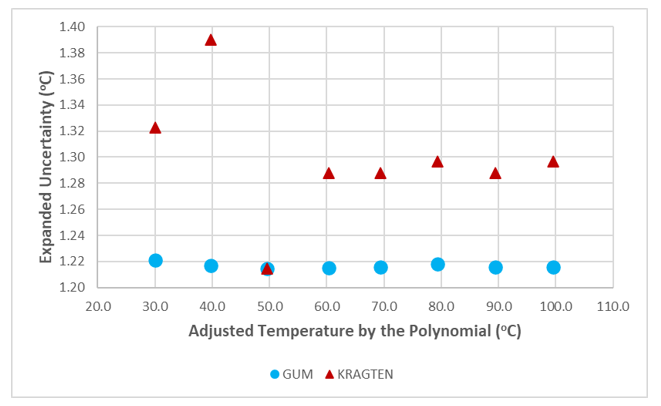Figure 1 Uncertainty of Measurement by methods: GUM and Kragten

Conclusion

In the industry, the importance of finding increasingly more minor uncertainties for the control of their measurement processes, specifically in the use of bimetallic thermometers for temperature control, is an activity that directly impacts the industry's economy. The consolidated results in this work allowed us to compare, from the metrological rigor, three methods for the evaluation of measurement uncertainty. The Relative Uncertainty method showed the lowest measurement uncertainty. However, it is not able to faithfully represent the physical nature of the problem. The explanation of this behavior that associates that the combination of relative uncertainties does not consider the mathematical calculation model of the measurand and should only be applied when it is defined by the multiplication and division of linear and independent terms. On the other hand, although Kragten's method is used to estimate uncertainty due to its mathematical robustness, it offers greater uncertainties when compared to the GUM method. Thus, the GUM method was more appropriate for estimating the measurement uncertainty associated with the temperature magnitude. Furthermore, it is noteworthy that the ordinary least squares method proved to be a suitable approach for: (i) deriving a second degree interpolating polynomial, which exhibits reduced uncertainty in comparison to the first-order polynomial typically utilized in industrial settings, and (ii) determining the temperature adjustment for any instrument reading within the calibration range. The methods applied in the investigation are valid for the physical principle of measurement associated with the thermal expansion between two metals. However, depending on the robustness of the methods developed, this study can be replicated for the evaluation of instruments that control other variables physical in the industry: pressure, dimensional and electrical measurements, among others.

Acknowledgement

To the Vicerrectoría de Ciencia, Tecnología e Innovación (VCTI) of the Universidad Antonio Nariño for the financing of Project No. 2022008 (February/2022 to December/2023) entitled: "Development of statistical techniques for the analysis of uncertainty and metrological performance in industrial equipment". In addition, this work was partially supported by the Ministerio de Ciencia, Tecnología e Innovación - Minciencias, in Colombia by  the project entitled “Gestión de energía basada en computación en la nube para la interoperabilidad entre un grupo de microrredes aisladas"  (70172) , which is part of  “Programa de Investigación en Tecnologías Emergentes para Microrredes Eléctricas Inteligentes con Alta Penetración de Energías Renovables" (10185270169).

Aro, R., Ayoub, M.W.B., Leito, I., Georgin, É., Savanier, B., 2021. Calibration and uncertainty estimation for water content measurement in solids. International Journal of Thermophysics, Volume 42(3), pp. 113

Cremona, P., Rogaume, T., Richard, F., Batiot, B., 2018. Application of the Kragten method in order to evaluate the uncertainty of the heat release rate determination using of the cone calorimeter. Journal of Physics: Conference Series, Volume 1107(3), p. 032019

Esraa, A., Putra, A., Mosa, A., Dan, R.M., Attia, O.H., 2022. An empirical model for optimizing the sound absorption of single layer MPP based on response surface methodology. International Journal of Technology, Volume 13(3), pp. 496507

Farrance, I., Badrick, T., Frenkel, R., 2018. Uncertainty in measurement: A review of the procedures for determining uncertainty in measurement and its use in deriving the biological variation of the estimated glomerular filtration rate. Practical Laboratory Medicine, Volume 12, p. e00097

Farrance, I., Frenkel, R., 2012. Uncertainty of measurement: a review of the rules for calculating uncertainty components through functional relationships. The Clinical Biochemist Reviews, Volume 33(2), 49–75

Gnauert, J., Jacobs, G., Kock, S., Bosse, D., 2018. Measurement uncertainty estimation of a novel torque transducer for wind turbine test benches. Journal of Physics: Conference Series, Volume 1065(4), p. 1065

Golijanek-J?drzejczyk, A., Mrowiec, A., Hanus, R., Zych, M., ?wisulski, D., 2019. Determination of the uncertainty of mass flow measurement using the orifice for different values of the Reynolds number. In: EPJ Web of Conferences, Volume 213, p.02022

Guerrasio, R., Haberhauer-Troyer, C., Steiger, M., Sauer, M., Mattanovich, D., Koellensperger, G., Hann, S., 2013. Measurement uncertainty of isotopologue fractions in fluxomics determined via mass spectrometry. Analytical and bioanalytical chemistry, Volume 405(15), pp. 51335146

Horsky, M., Irrgeher, J., Prohaska, T., 2016. Evaluation strategies and uncertainty calculation of isotope amount ratios measured by MC ICP-MS on the example of Sr. Analytical and Bioanalytical Chemistry, Volume 408(2), 351367

International Organization for Standardization, 2015. ISO 9001:2015. Quality management systems requirements. European Committee for Standardization, Brussels

Joint Committee for Guides in Metrology (JCGM), 2008. Evaluation of measurement data—Guide to the expression of uncertainty in measurement. Bureau International des Poids et Mesures JCGM 100:2008, pp. 1–134

Joint Committee for Guides in Metrology (JCGM), 2012. The international vocabulary of metrology—basic and general concepts and associated terms (VIM). Joint Committee for Guides in Metrology (JCGM)

Khan, S.G., Ibrayeva, L.K., 2020. Analysis of methods for estimating the uncertainty of the relative error of electromagnetic flowmeters reservoirs., Physics AUC, Volume 30, pp. 115

Sardjono, H., Wijonarko, S., 2018. Calibration process quantity reduction of the thermal voltage converter standard using a three-stage build-up and build-down method. International Journal of Technology, Volume 9(1), pp. 181191

Theodorou, D., Zannikou, Y., Zannikos, F., 2012. Estimation of the standard uncertainty of a calibration curve: application to sulfur mass concentration determination in fuels. Accreditation and Quality Assurance, Volume 17(3), pp. 275281

Wang, C., Caja, J., Gómez, E., 2018. Comparison of methods for outlier identification in surface characterization. Measurement, Volume 117, pp. 312325

Wang, J., Wang, Z., de-Dear, R., Luo, M., Ghahramani A., Lin, B., 2018. The uncertainty of subjective thermal comfort measurement. Energy and Buildings, Volume 181, pp. 3849

Zárate, D.M., Cárdenas, F., Forero, E.F., Peña, F.O., 2022. Strength of concrete through ultrasonic pulse velocity and uniaxial compressive strength. International Journal of Technology, Volume 13(1), pp. 103114